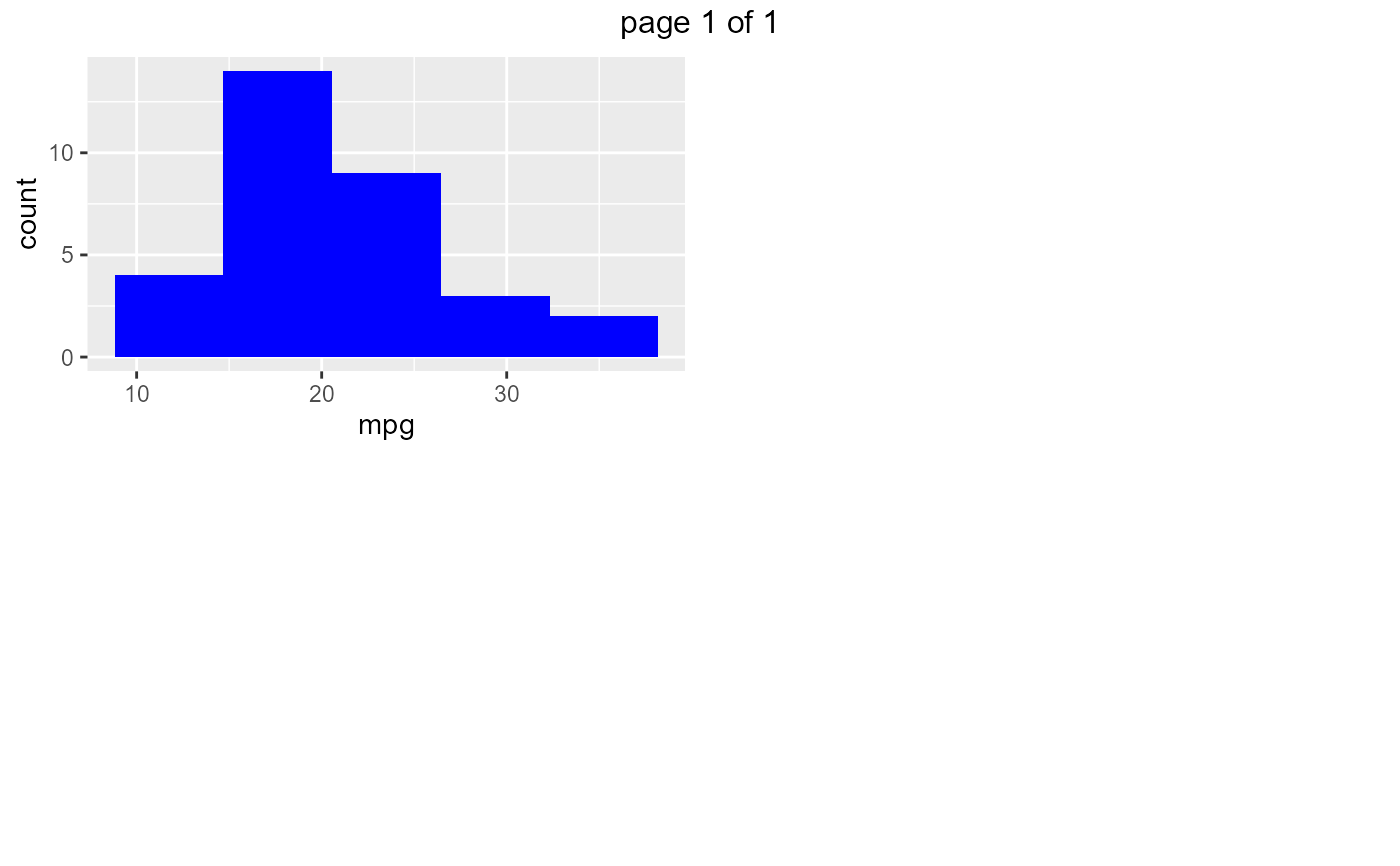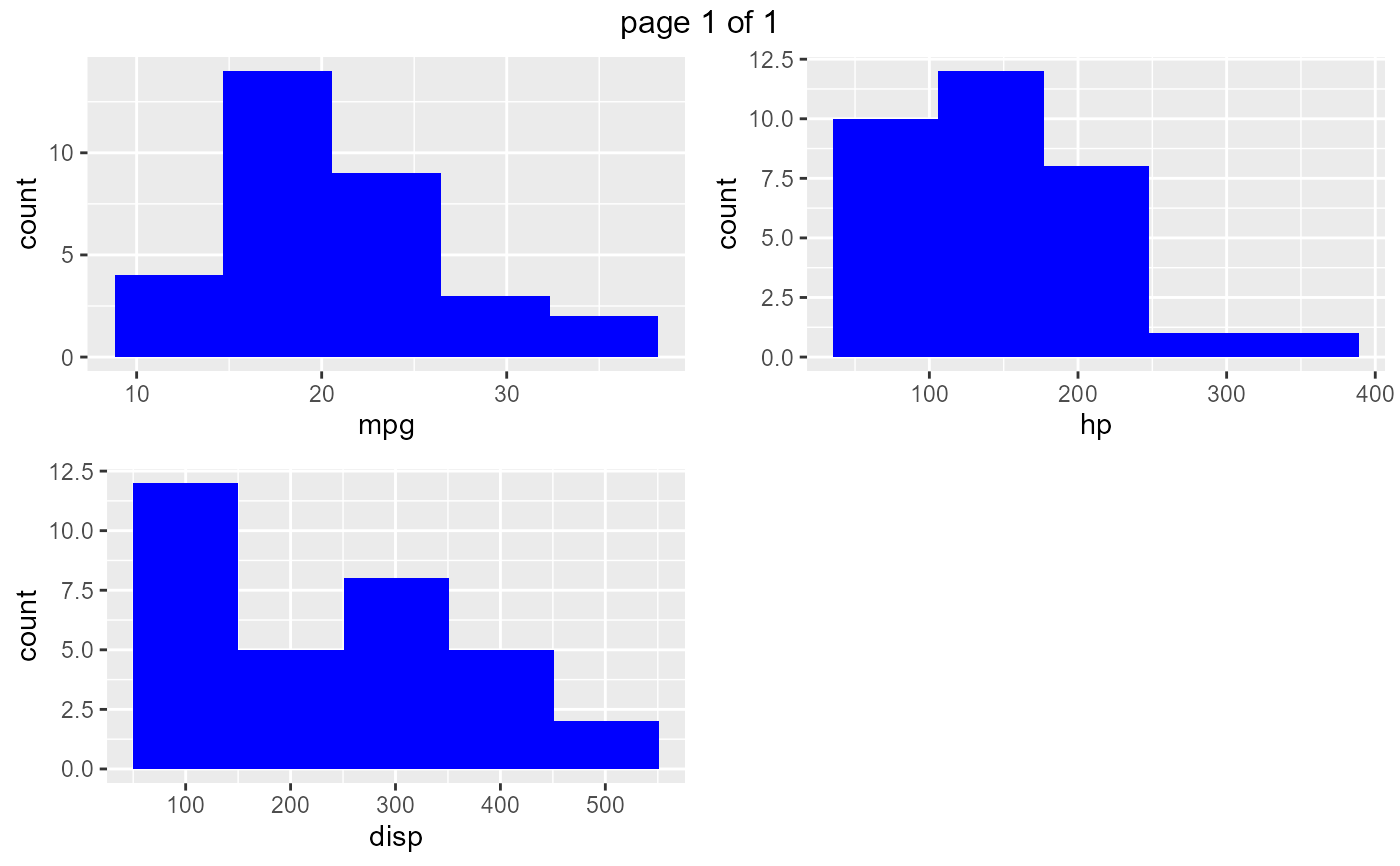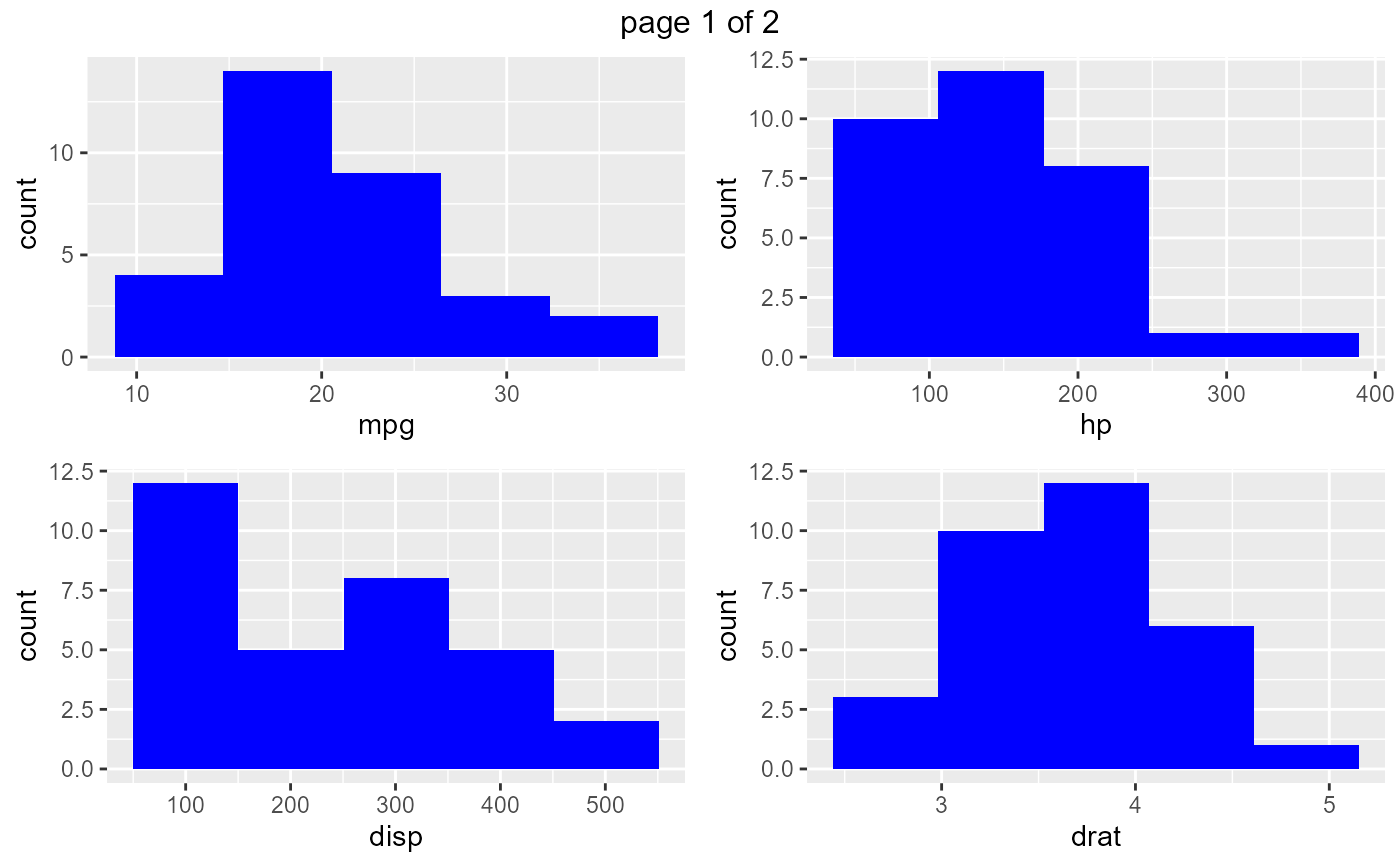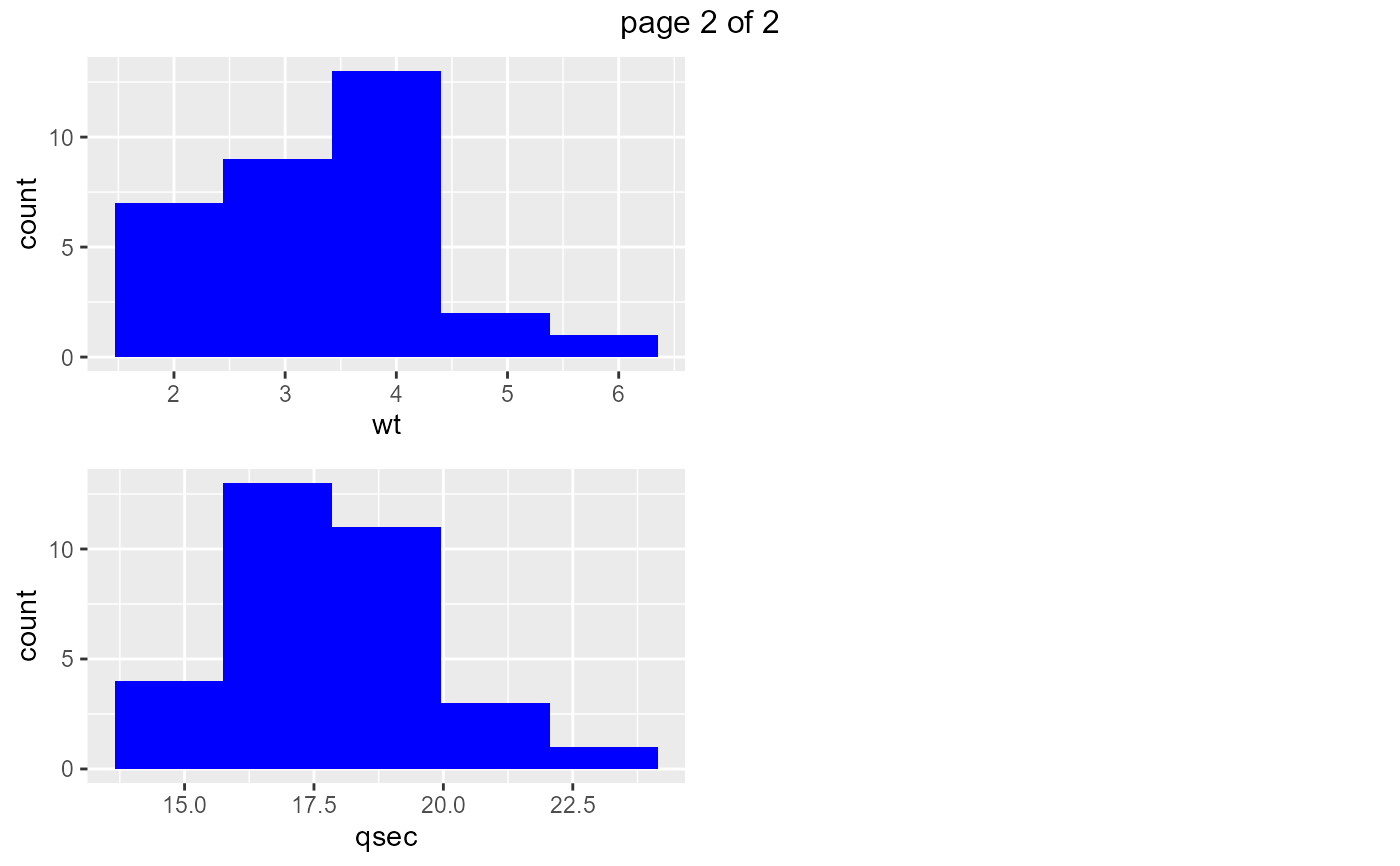Creates histograms if the data has continuous variables.

ds_plot_histogram(data, ..., bins = 5, fill = "blue", print_plot = TRUE)

## Arguments

data A data.frame or tibble. Column(s) in data. Number of bins in the histogram. Color of the histogram. logical; if TRUE, prints the plot else returns a plot object.

## Examples

# plot single variable
ds_plot_histogram(mtcarz, mpg)# plot multiple variables
ds_plot_histogram(mtcarz, mpg, disp, hp)# plot all variables
ds_plot_histogram(mtcarz)